JEE  >  Test: Single Correct MCQs: Permutations and Combinations | JEE Advanced

# Test: Single Correct MCQs: Permutations and Combinations | JEE Advanced

Test Description

## 15 Questions MCQ Test Mathematics For JEE | Test: Single Correct MCQs: Permutations and Combinations | JEE Advanced

Test: Single Correct MCQs: Permutations and Combinations | JEE Advanced for JEE 2023 is part of Mathematics For JEE preparation. The Test: Single Correct MCQs: Permutations and Combinations | JEE Advanced questions and answers have been prepared according to the JEE exam syllabus.The Test: Single Correct MCQs: Permutations and Combinations | JEE Advanced MCQs are made for JEE 2023 Exam. Find important definitions, questions, notes, meanings, examples, exercises, MCQs and online tests for Test: Single Correct MCQs: Permutations and Combinations | JEE Advanced below.
Solutions of Test: Single Correct MCQs: Permutations and Combinations | JEE Advanced questions in English are available as part of our Mathematics For JEE for JEE & Test: Single Correct MCQs: Permutations and Combinations | JEE Advanced solutions in Hindi for Mathematics For JEE course. Download more important topics, notes, lectures and mock test series for JEE Exam by signing up for free. Attempt Test: Single Correct MCQs: Permutations and Combinations | JEE Advanced | 15 questions in 30 minutes | Mock test for JEE preparation | Free important questions MCQ to study Mathematics For JEE for JEE Exam | Download free PDF with solutions
 1 Crore+ students have signed up on EduRev. Have you?
Test: Single Correct MCQs: Permutations and Combinations | JEE Advanced - Question 1

### nCr–1 = 36, nCr = 84 and nCr + 1 = 126, then r is : (1979)

Detailed Solution for Test: Single Correct MCQs: Permutations and Combinations | JEE Advanced - Question 1

nCr -1 = 36, nCr = 84,nCr+1= 126
KEY CONCEPT : We know that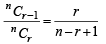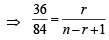⇒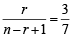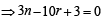....(1)

⇒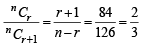⇒ 2n – 5r – 3 = 0 ....(2)

Solving (1) and (2), we get n = 9 and r = 3.

Test: Single Correct MCQs: Permutations and Combinations | JEE Advanced - Question 2

### Ten different letters of an alphabet are given. Words with five letters are formed from these given letters. Then the number of words which have at least one letter repeated are (1982 - 2 Marks)

Detailed Solution for Test: Single Correct MCQs: Permutations and Combinations | JEE Advanced - Question 2

Total number of words that can be formed using 5 letters out of 10 given different letters
= 10 × 10 × 10 × 10 × 10 (as letters can repeat)
= 1, 00, 000
Number of words that can be formed using 5 different letters out of 10 different letters
= 10P5 (none can repeat)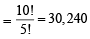∴ Number of words in which at least one letter is repeated
= total words–words with none of the letters repeated
= 1,00,000 – 30,240
= 69760

Test: Single Correct MCQs: Permutations and Combinations | JEE Advanced - Question 3

### The value of the  expression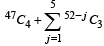is equal to (1982 - 2 Marks)

Detailed Solution for Test: Single Correct MCQs: Permutations and Combinations | JEE Advanced - Question 3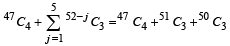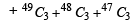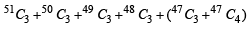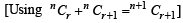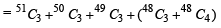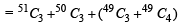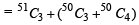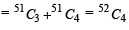Test: Single Correct MCQs: Permutations and Combinations | JEE Advanced - Question 4

Eight chairs are numbered 1 to 8. Two women and three men wish to occupy one chair each. First the women choose the chairs from amongst the chairs marked 1 to 4 ; and then the men select the chairs from amongst the remaining. The number of possible arrangements is (1982 - 2 Marks)

Detailed Solution for Test: Single Correct MCQs: Permutations and Combinations | JEE Advanced - Question 4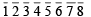Two women can choose two chairs out of 1, 2, 3, 4, in 4C2 ways and can arrange themselves in 2! ways.
Three men can choose 3 chairs out of 6 remaining chairs in 6C3 ways and can arrange themselves in 3! ways
∴ Total number of possible arrangements are
4C2× 2! × 6C3 × 3!=4P2× 6P3

Test: Single Correct MCQs: Permutations and Combinations | JEE Advanced - Question 5

A five-digit numbers divisible by 3 is to be formed using the numerals 0, 1, 2, 3, 4 and 5, without repetition. The total number of ways this can be done is (1989 - 2 Marks)

Detailed Solution for Test: Single Correct MCQs: Permutations and Combinations | JEE Advanced - Question 5

KEY CONCEPT : We know that a number is divisible by 3 if the sum of its digits is divisibly by 3.
Now out of  0, 1, 2, 3, 4, 5 if we take 1, 2, 3, 4, 5 or 0, 1, 2, 4, 5 then the 5digit numbers will be divisible by 3.
Case I : Number of 5 digit numbers formed using the
digits 1, 2, 3, 4, 5 = 5! = 120
Case II : Taking 0, 1, 2, 4, 5 if we make 5 digit number then
I place can be filled in = 4 ways (0 can not come at I place)
II place can be filled in = 4 ways
III place can be filled in = 3 ways
IV place can be filled in = 2 ways
V place can be filled in = 1 ways
∴ Total numbers are = 4 × 4! = 96
Thus total numbers divisible by 3 are
= 120 + 96 = 216

Test: Single Correct MCQs: Permutations and Combinations | JEE Advanced - Question 6

How many different nine digit numbers can be formed from the number 223355888 by rearranging its digits so that the odd digits occupy even positions ? (2000S)

Detailed Solution for Test: Single Correct MCQs: Permutations and Combinations | JEE Advanced - Question 6

X – X – X – X – X. The four digits 3, 3, 5, 5 can be arranged at (–) places in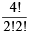= 6 ways.

The five digits 2, 2, 8, 8, 8 can be arranged at

(X) places in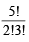= 10ways.

Total no. of arrangements = 6 × 10 = 60 ways.

Test: Single Correct MCQs: Permutations and Combinations | JEE Advanced - Question 7

Let Tn denote the number of triangles which can be formed using the vertices of a regular polygon of n sides. If Tn + 1 - Tn = 21, then n equals (2001S)

Detailed Solution for Test: Single Correct MCQs: Permutations and Combinations | JEE Advanced - Question 7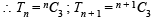As per question,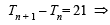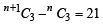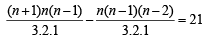⇒ n(n - 1) (n + 1 -n + 2)= 126
⇒ n (n – 1) = 42 ⇒ n (n – 1) = 7 × 6  ⇒ n = 7.

Test: Single Correct MCQs: Permutations and Combinations | JEE Advanced - Question 8

The number of arrangements of the letters of the word BANANA in which the two N's do not appear adjacently is (2002S)

Detailed Solution for Test: Single Correct MCQs: Permutations and Combinations | JEE Advanced - Question 8

Total number of ways of arranging the letters of the word BANANA is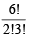= 60 Number of words in which 2 N’s come together is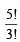= 20

Hence the required number = 60 – 20  = 40

Test: Single Correct MCQs: Permutations and Combinations | JEE Advanced - Question 9

A rectangle with sides of length (2m – 1) an d (2n – 1) units is divided into squares of unit length by drawing parallel lines as shown in the diagram, then the number of rectangles possible with odd side length s is (2005S)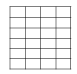Detailed Solution for Test: Single Correct MCQs: Permutations and Combinations | JEE Advanced - Question 9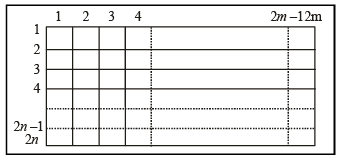If we see the blocks in terms of lines then there are 2m vertical lines and 2n horizontal lines. To form the required rectangle we must select two horizontal lines, one even numbered (out of 2, 4, .....2n) and one odd numbered (out of 1, 3....2n–1) and similarly two vertical lines. The number of rectangles is
mC1 .  mC1 . nC1 . nC1 = m2n2

Test: Single Correct MCQs: Permutations and Combinations | JEE Advanced - Question 10

If the LCM of p, q is r2t4s2, where r, s, t are prime numbers and p, q are the positive integers then the number of ordered pair (p, q) is (2006 - 3M, –1)

Detailed Solution for Test: Single Correct MCQs: Permutations and Combinations | JEE Advanced - Question 10

∵ r, s, t are prime numbers,
∴  Section of (p, q) can be done as follows

∴ r can be selected 1 + 1 + 3 = 5 ways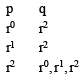Similarly s and t can be selected in 9 and 5 ways respectivley.
∴ Total ways = 5 × 9 × 5 = 225

Test: Single Correct MCQs: Permutations and Combinations | JEE Advanced - Question 11

The letters of the word COCHIN are permuted and all the permutations are arranged in an alphabetical order as in an English dictionary. The number of words that appear before the word COCHIN is (2007 -3 marks)

Detailed Solution for Test: Single Correct MCQs: Permutations and Combinations | JEE Advanced - Question 11

The letter of word COCHIN in alphabetic order are C, C, H, I, N, O.
Fixing first letter C and keeping C at second place, rest 4 can be arranged in 4! ways.
Similarly the words starting with CH, CI, CN are 4! in each case.
Then fixing first two letters as CO next four places when filled in alphabetic order give  the word COCHIN.
∴ Numbers of words coming before COCHIN are
4 × 4! = 4 × 24 = 96

Test: Single Correct MCQs: Permutations and Combinations | JEE Advanced - Question 12

The number of seven digit i ntegers, with sum of the digits equal to 10 and formed by using the digits 1, 2 and 3 only, is (2009)

Detailed Solution for Test: Single Correct MCQs: Permutations and Combinations | JEE Advanced - Question 12

We h ave to for m 7 digit n umber s, using th e digits 1, 2 and 3 only, such that the sum of the digits in a number = 10.
This can be done by taking 2, 2, 2, 1, 1, 1, 1, or by taking 2, 3, 1, 1, 1, 1, 1.
∴ Number of ways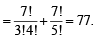Test: Single Correct MCQs: Permutations and Combinations | JEE Advanced - Question 13

The total number of ways in which 5 balls of different colours can be distributed among 3 persons so that each person gets at least one ball is     (2012)

Detailed Solution for Test: Single Correct MCQs: Permutations and Combinations | JEE Advanced - Question 13

∵ Each person gets at least one ball.
∴ 3 Per son s can have 5 balls in th e followin g systems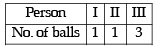or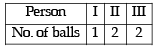The number of ways to distribute the balls in first system =
5C1 x 4C1 x 3C3

Also 3, persons having 1, 1 and 3 balls can be arranged in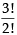ways.

∴ No. of ways to distribute 1, 1, 3 balls to the three persons

5C1 x 4C1 x 3C3 x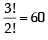Similarly the total no. of ways to distribute 1, 2, 2 balls to the three persons = 5C1 x 4C2 x 2C2 x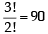∴ The required number of ways = 60 + 90 = 150

Test: Single Correct MCQs: Permutations and Combinations | JEE Advanced - Question 14

Six cards and six envelopes are numbered 1, 2, 3, 4, 5, 6 and cards are to be placed in envelopes so that each envelope contains exactly one card and no card is placed in the envelope bearing the same number and moreover the card numbered 1 is always placed in envelope numbered 2. Then the number of ways it can be done is (JEE Adv. 2014)

Detailed Solution for Test: Single Correct MCQs: Permutations and Combinations | JEE Advanced - Question 14

∵ Car d numbered 1 is always placed in envelope numbered 2, we can consider two cases.
Case I: Card numbered 2 is placed in envelope numbered 1.
Then it is dearrangement of 4 objects, which can be done in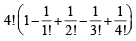= 9 ways

Case II: Card numbered 2 is not placed in envelope numbered 1.
Then it is dearrangement of 5 objects, which can be done in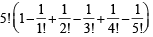= 44 ways

∴ Total ways = 44 + 9 = 53

Test: Single Correct MCQs: Permutations and Combinations | JEE Advanced - Question 15

A debate club consists of 6 girls and 4 boys. A team of 4 members is to be selected from this club including the selection of a captain (from among these 4 memoers) for the team. If the team has to include at most one boy, then the number of ways of selecting the team is (JEE Adv. 2016)

Detailed Solution for Test: Single Correct MCQs: Permutations and Combinations | JEE Advanced - Question 15

Either one boy will be selected or no boy will be selected. Also out of four members one captain is to be selected.
∴ Required number of ways = (4C1 × 6C3+ 6C4) × 4C1 = (80 + 15) × 4 = 380

## Mathematics For JEE

130 videos|359 docs|306 tests
 Use Code STAYHOME200 and get INR 200 additional OFF Use Coupon Code
Information about Test: Single Correct MCQs: Permutations and Combinations | JEE Advanced Page
In this test you can find the Exam questions for Test: Single Correct MCQs: Permutations and Combinations | JEE Advanced solved & explained in the simplest way possible. Besides giving Questions and answers for Test: Single Correct MCQs: Permutations and Combinations | JEE Advanced, EduRev gives you an ample number of Online tests for practice

## Mathematics For JEE

130 videos|359 docs|306 tests